# ML Aggarwal Solutions for Class 7 Maths Chapter 10 - Lines and Angles

ML Aggarwal Solutions for Class 7 Maths Chapter 10 – Lines and Angles are provided here in a step by step manner to help students grasp the concepts easily and score well in their exams. This chapter mainly deals with problems based on lines and angles. For a better understanding of the concepts, students can solve the problems using the ML Aggarwal Solutions, which are designed by our expert faculty team at BYJU’S with utmost care. Students aspiring to secure high marks in their board examination are advised to practice the solutions on a regular basis.

Chapter 10 – Lines and Angles provides solutions to questions related to each and every topic present in this chapter. Students can refer to ML Aggarwal Class 7 Solutions pdf and can easily download for free from the links given below and can use it for future reference, as well.

## Download the Pdf of ML Aggarwal Solutions for Class 7 Maths Chapter 10 – Lines and Angles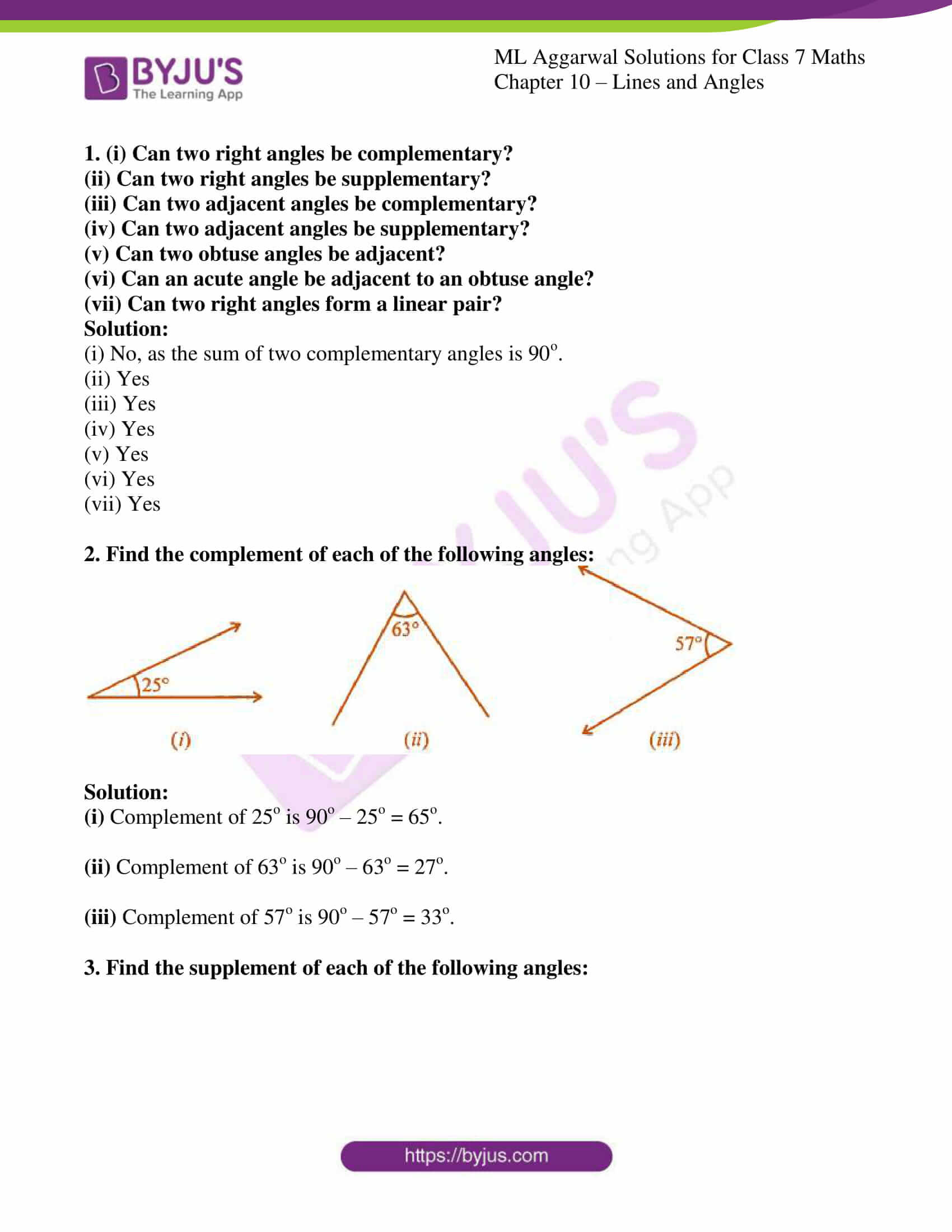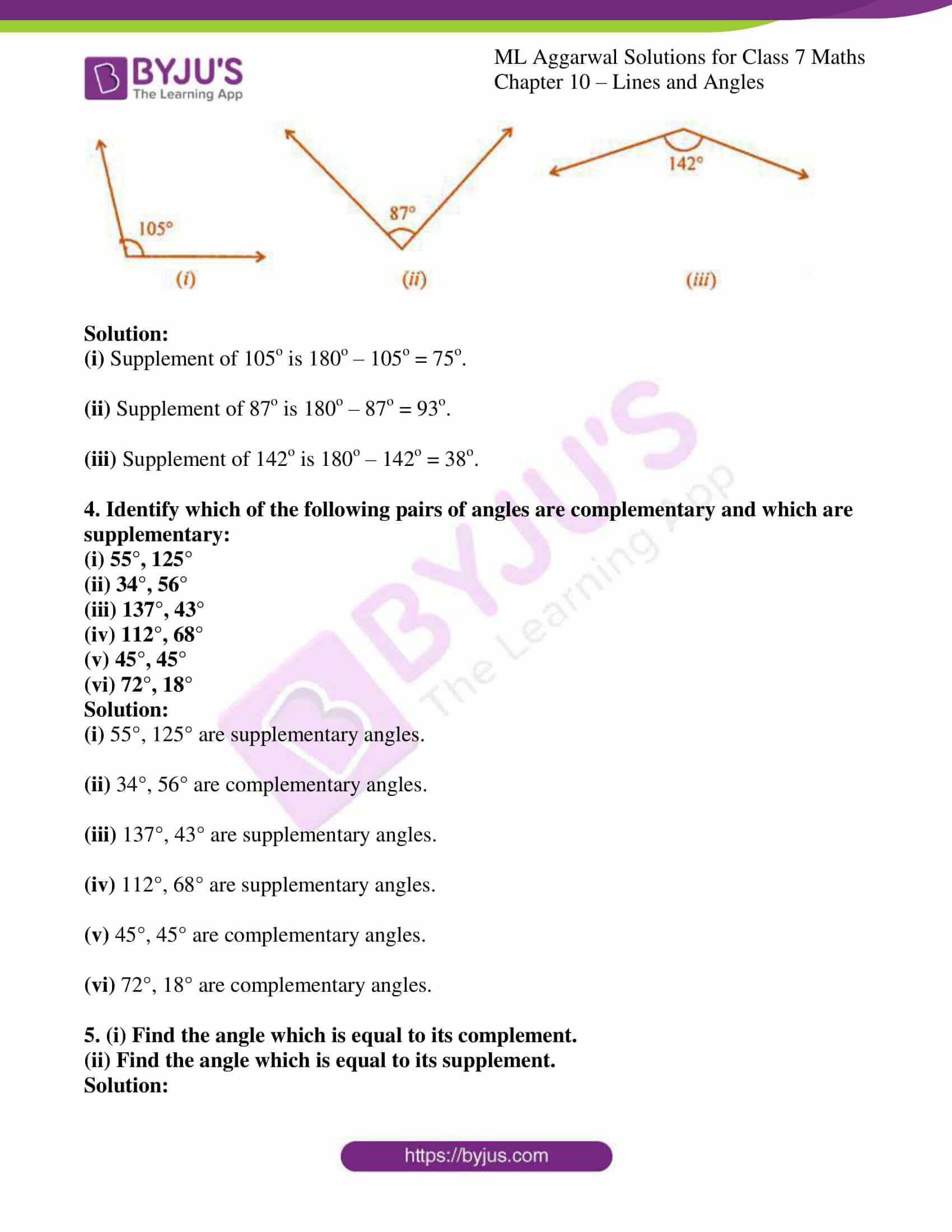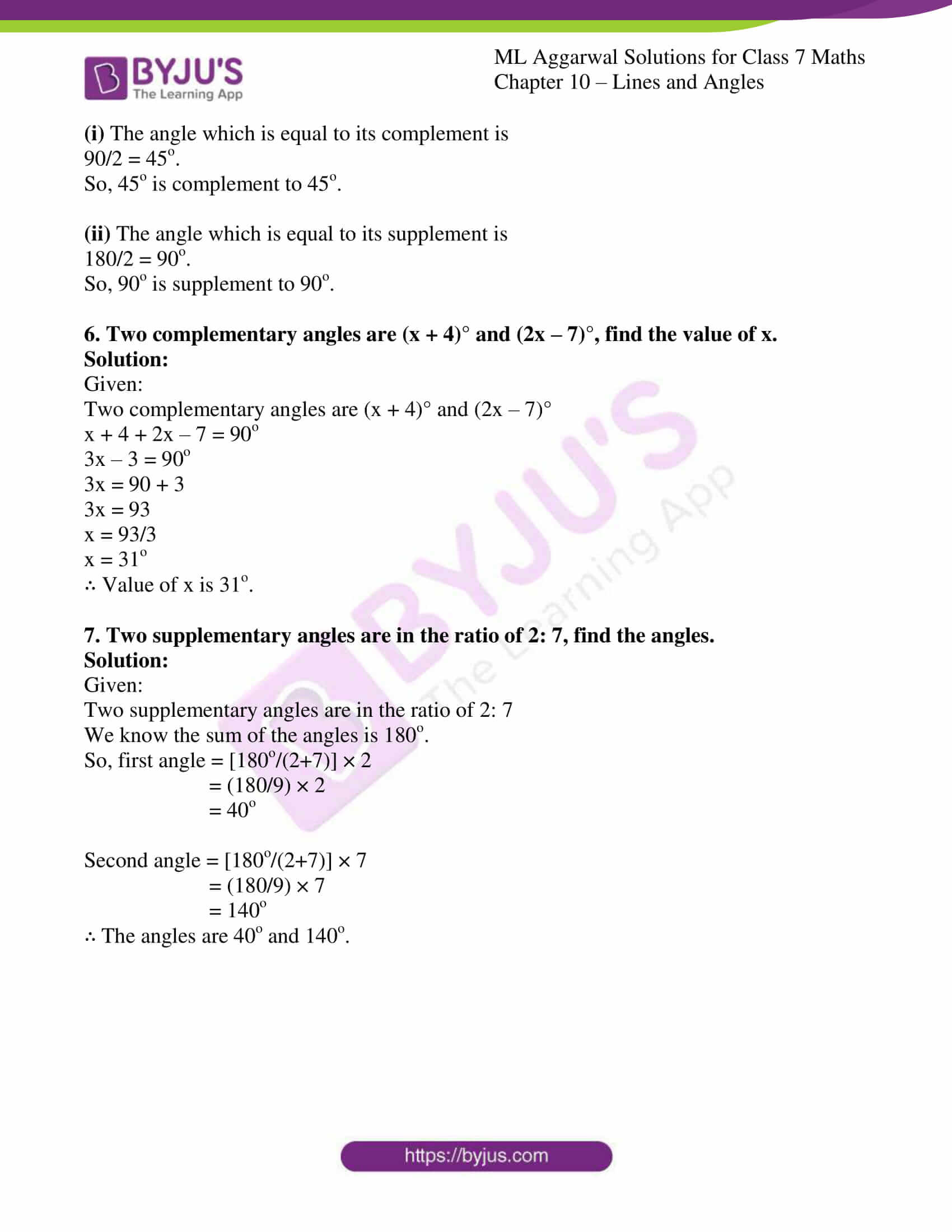### Access answers to ML Aggarwal Solutions for Class 7 Maths Chapter 10 – Lines and Angles

1. (i) Can two right angles be complementary?

(ii) Can two right angles be supplementary?

(iii) Can two adjacent angles be complementary?

(iv) Can two adjacent angles be supplementary?

(v) Can two obtuse angles be adjacent?

(vi) Can an acute angle be adjacent to an obtuse angle?

(

vii) Can two right angles form a linear pair?

Solution:

(i) No, as the sum of two complementary angles is 90o.

(ii) Yes

(iii) Yes

(iv) Yes

(v) Yes

(vi) Yes

(vii) Yes

2. Find the complement of each of the following angles: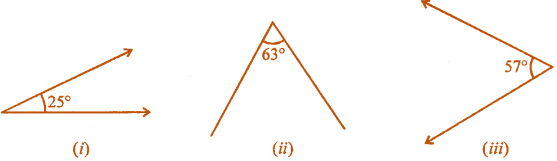Solution:

(i) Complement of 25o is 90o – 25o = 65o.

(ii) Complement of 63o is 90o – 63o = 27o.

(iii) Complement of 57o is 90o – 57o = 33o.

3. Find the supplement of each of the following angles: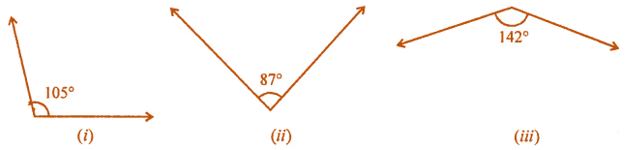Solution:

(i) Supplement of 105o is 180o – 105o = 75o.

(ii) Supplement of 87o is 180o – 87o = 93o.

(iii) Supplement of 142o is 180o – 142o = 38o.

4. Identify which of the following pairs of angles are complementary and which are supplementary:
(i) 55°, 125°
(ii) 34°, 56°
(iii) 137°, 43°
(iv) 112°, 68°
(v) 45°, 45°
(vi) 72°, 18°
Solution:

(i) 55°, 125° are supplementary angles.

(ii) 34°, 56° are complementary angles.

(iii) 137°, 43° are supplementary angles.

(iv) 112°, 68° are supplementary angles.

(v) 45°, 45° are complementary angles.

(vi) 72°, 18° are complementary angles.

5. (i) Find the angle which is equal to its complement.
(ii) Find the angle which is equal to its supplement.
Solution:

(i) The angle which is equal to its complement is

90/2 = 45o.

So, 45o is complement to 45o.

(ii) The angle which is equal to its supplement is

180/2 = 90o.

So, 90o is supplement to 90o.

6. Two complementary angles are (x + 4)° and (2x – 7)°, find the value of x.
Solution:

Given:

Two complementary angles are (x + 4)° and (2x – 7)°

x + 4 + 2x – 7 = 90o

3x – 3 = 90o

3x = 90 + 3

3x = 93

x = 93/3

x = 31o

∴ Value of x is 31o.

7. Two supplementary angles are in the ratio of 2: 7, find the angles.
Solution:

Given:

Two supplementary angles are in the ratio of 2: 7

We know the sum of the angles is 180o.

So, first angle = [180o/(2+7)] × 2

= (180/9) × 2

= 40o

Second angle = [180o/(2+7)] × 7

= (180/9) × 7

= 140o

∴ The angles are 40o and 140o.